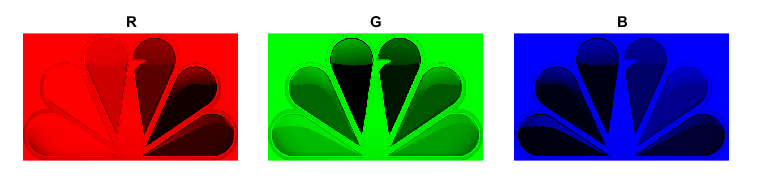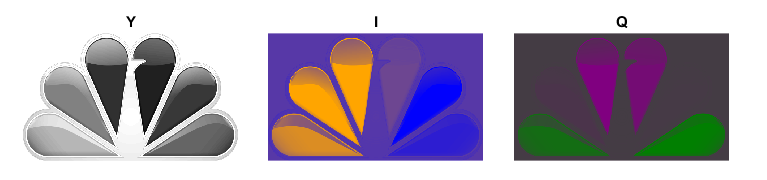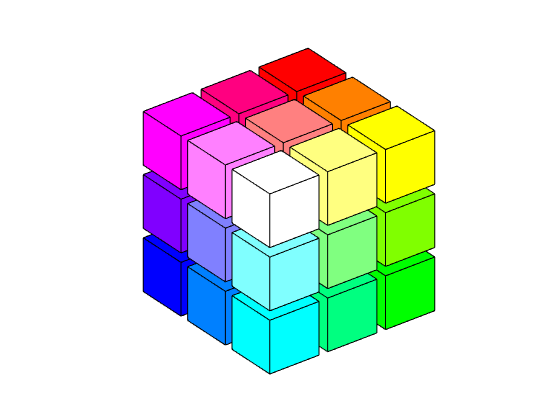# My First Matrix, RGB, YIQ, and Color Cubes

When I was in high school in the 1950's, I didn't know anything about matrices. But I nearly encountered one when I wrote a paper for my physics class about the color scheme that allowed new color TV broadcasts to be compatible with existing black-and-white TV receivers.

### Contents

#### KTVT

During my high school years in Utah, I worked part-time and summers as a camera man and projectionist at what was then called KTVT, Channel 4, and the local NBC affiliate in Salt Lake City. The TV cameras at the time employed vacuum tubes, not transistors. They were the size of suit cases, weighed over 100 pounds, and were mounted on dollies.

The first coast-to-coast TV broadcast in color was the Tournament of Roses parade on January 1, 1954. KTVT had a prototype color receiver, perhaps the only one in Utah at the time. We set up bleachers in the studio and invited guests to see the new technology.

The NTSC compatible color television standard, adopted in 1953, allows color information to be encoded in a broadcast in such a way that existing black-and-white receivers will still function without modification. The standard remained in use in the US until broadcasters ceased analog transmissions in 2009.

#### High School Physics

I wrote a paper for my high school physics class about the NTSC compatible color scheme. It involves a luminance-chrominance conversion between the RGB, red-green-blue, signal used in color studio equipment and home receivers, and a YIQ encoding. Y represents the brightness, or luma, of the signal, and is used by itself for black-and-white. The letters I and Q stand for in-phase and quadrature. These two components combine with the brightness to produce color.

Here are the conversion formulas that I had in my high school paper.

$$Y = 0.2989 R + 0.5870 G + 0.1140 B$$

$$I = 0.5959 R - 0.2744 G - 0.3216 B$$

$$Q = 0.2115 R - 0.5229 G + 0.3114 B$$

#### The Matrix

I now know how to write the conversion formulas in matrix notion. If $x$ is the 3-vector containing RGB and $y$ is the 3-vector of YIQ, then

$$y = A x$$

where $A$ is the matrix

$$A = \left[ \begin{array}{rrr} 0.2989 & 0.5870 & 0.1140 \\ 0.5959 & -0.2744 & -0.3216 \\ 0.2115 & -0.5229 & 0.3114 \end{array} \right]$$

In retrospect, this was my very first matrix.

Of course, conversion at the receiving end from YIQ to RGB is done by

$$x = A^{-1} y$$

#### MATLAB

Fast forward 25 years so we can use MATLAB.

   A = [0.2989  0.5870  0.1140
0.5959 -0.2744 -0.3216
0.2115 -0.5229  0.3114]

T = inv(A)

A =
0.2989    0.5870    0.1140
0.5959   -0.2744   -0.3216
0.2115   -0.5229    0.3114
T =
1.0002    0.9560    0.6211
1.0001   -0.2720   -0.6470
1.0000   -1.1060    1.7030


The first column of $A^{-1}$ is all 1's, so each of R, G and B gets a full dose of Y.

#### Colorspaces

Although I had nothing to do with putting them there, these two matrices are central to the functions ntsc2rgb and rgb2ntsc in the colorspaces folder of the Image Processing Toolbox. In the following code A is a 3D full color RGB image.

Here is the core of the NTSC to RGB function. The image is reshaped to a tall, skinny 2D array and the transformation applied on the right by the transpose of T.

   dbtype 36:36 ntsc2rgb
dbtype 41:43 ntsc2rgb
dbtype 48:48 ntsc2rgb

36    T = [1.0 0.956 0.621; 1.0 -0.272 -0.647; 1.0 -1.106 1.703];

41        m = size(A,1);
42        n = size(A,2);
43        A = reshape(A(:),m*n,3)*T';

48        A = reshape(A,m,n,3);


The conversion from RGB to NTSC uses matrix division on the right by T transpose. This may be the only place in MATLAB where the forward slash matrix operator appears.

   dbtype 30:31 rgb2ntsc
dbtype 35:35 rgb2ntsc

30    T = [1.0 0.956 0.621; 1.0 -0.272 -0.647; 1.0 -1.106 1.703].';
31    [so(1),so(2),thirdD] = size(A);

35        A = reshape(reshape(A,so(1)*so(2),thirdD)/T,so(1),so(2),thirdD);


#### Grayscale

Since analog NTSC broadcasts are history, these conversions are probably not very common any more. But the single formula

$$Y = 0.2989 R + 0.5870 G + 0.1140 B$$

is the basis for the rgb2gray function.

#### NBC Peacock

NBC introduced a peacock logo to promote the debut of its "in living color" broadcasts. The peacock has lost a few feathers over the years, but it still provides an excellent color test pattern.(NBC SVG by FOX 52 [Public domain], via Wikimedia Commons)

Here are the red, green and blue components. The bright portions represent high values. All three have relatively bright backgrounds and bright peacocks in the center; these combine to produce white. The R component has bright feathers on the left to contribute to the yellow, orange and red feathers in the full logo. The G component has bright components on the bottom to contribute to the yellow and green in the full. And B has bright feathers on the upper right to give blue and purple.Convert to YIQ. The Y component is the grayscale rendition to drive old black-and-white TVs. The I component goes from orange to blue and the Q component goes from purple to green.#### Colorcubes

Talking about color, here is one of my favorite graphics. Let's start with an animated .gif of colorcubes(3), which contains 27 cubelets. If you run colorcubes yourself, you will drive the rotation with your mouse.

You can see white at one vertex and black at the opposite vertex. Red, a bright green, and blue are at three vertices, while their complements -- cyan, magenta, and yellow -- oppose them. Actually, the green vertex is too bright. It is often called "lime". A darker green is along the bottom edge, halfway between lime and black. Here's a little quiz: What color is the hidden cubelet in the center?Now a look at the construction of colorcubes(5).#### Colorcubes Code

Here is the colorcubes code.

   type colorcubes

function colorcubes(n,w)
% COLORCUBES  A cube of cubes in the RGB color space.
%   COLORCUBES, with no arguments, shows 5^3 = 125 cubes with
%      colors equally spaced in the RGB color space.
%   COLORCUBES(n) shows n-by-n-by-n colors.
%   COLORCUBES(2) shows 8 colors: R, G, B, C, M, Y, W, K (black).
%   COLORCUBES(n,w) uses cubes of width w.  Default is w = 0.85.
%   Rotate the cube with the mouse or arrow keys.

%   Copyright 2016 The MathWorks, Inc.

if nargin < 1, n = 5; end
if nargin < 2, w = 0.85; end
initgraphics(n)
[x,y,z] = cube(w);
m = n-1;
for i = m:-1:0
for j = m:-1:0
for k = 0:m
r = k/m;
g = 1-j/m;
b = 1-i/m;
surface(i+x,j+y,k+z, ...
'facecolor',[r g b], ...
'facelighting','gouraud');
drawnow
end %k
end %j
end %i

% ------------------------

% INITGRAPHCS  Inialize the colorcubes axis.
%   INITGRAPHICS(n) for n-by-n-by-n display.

function initgraphics(n)
clf reset
shg
set(gcf,'color','white')
axis([0 n 0 n 0 n]);
axis off
axis vis3d
rotate3d on
end %initgraphics

function [x,y,z] = cube(w)
% CUBE  Coordinates of the faces of a cube.
%   [x,y,z] = cube(w); surface(x,y,z)
%   plots a cube of with w.

u = [0 0; 0 0; w w; w w];
v = [0 w; 0 w; 0 w; 0 w];
z = [w w; 0 0; 0 0; w w];
s = [nan nan];
x = [u; s; v];
y = [v; s; u];
z = [z; s; w-z];
end %cube

end % colorcubes



Published with MATLAB® R2016a

|

### コメント

コメントを残すには、ここ をクリックして MathWorks アカウントにサインインするか新しい MathWorks アカウントを作成します。